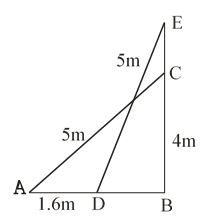#### A 5 m long ladder is placed leaning towards a vertical wall such that it reaches the wall at a point 4 m high. If the foot of the ladder is moved 1.6 m towards the wall, then find the distance by which the top of the ladder would slide upwards on the wall.Here,   AC = 5m, BC = 4 m, AD = 1.6 m

Let       CE = x m

In  use Pythagoras theorem

Similarly, in triangle EBD use Pythagoras theorem

Hence the top of the ladder would slide up words on the wall at distance is 0.8 m.